This page has been robot translated, sorry for typos if any. Original content here.

# EXPERIENCE OF IDENTIFICATION OF UNDER ACCOUNT OF ELECTRIC ENERGY

Electricity metering is an integral part of any enterprise. First of all, it is necessary for the implementation of monetary settlement between the energy supplying organization and consumers. The main goal of commercial electricity metering is to obtain reliable information about the amount of consumed (issued) electric energy. In three-phase four-wire systems, for measuring the consumed electric energy, three-element energy meters are used, which are a measuring wattmeter system and belong to integrating devices. An energy meter is a device that responds not only to the value of energy, but also to the direction of its transmission, therefore, when connecting the meter, it is necessary to ensure both the correct directions of the currents and their correct combination with the voltages in each element of the meter. Active energy measured by the meter for time t 2 - t 1 is defined as: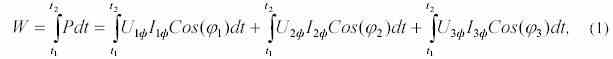where the index "f" denotes phase voltages and currents. Let us determine the amount of under-accounting of electricity for some of the most common failures of the elements of the measuring complex of electricity metering and errors in connecting electricity meters.

Open circuit or secondary winding of a current transformer (CT). The current in the secondary circuit is zero, suppose in the phase indicated by the index "1" (I 1ph = 0). With a symmetrical load, the active energy measured by the meter, according to (1):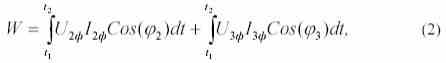those. will be 2/3 of the actual value. The same amount of underestimation is observed in the absence of voltage on one of the meter elements (on the parallel meter winding). The marking in the current circuits is mixed up , as a result of which the wires coming from the CT are interchanged, let us say the phases with the index "2". In this case, the current flows in all phases, but in the phase "2" has the opposite direction. In other words, in the vector diagram (Fig. 1), the current of phase “2” is rotated 180 0 , therefore, the energy taken into account by the meter: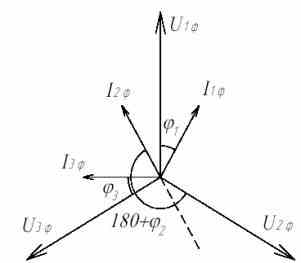Figure 1. Vector diagram of currents and voltages on the meter when connecting CT phase "2" with reverse polarity at an active inductive load.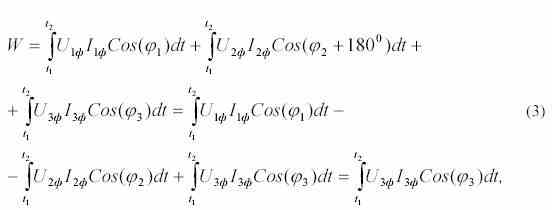those. with a symmetrical load will be 1/3 of the actual value.

To check the correct connection of the meter, it is enough to remove the vector diagram and build it, compare it with the expected one, which depends on the nature of the load. Vector diagrams are most convenient to shoot with VAF-85M or VAF-A devices. To obtain a reliable measurement result, the vector diagram should be taken directly from the meter terminals, which are usually located under the seal of the power supply organization, so you can use a simplified verification method, for which you need to: measure all phase voltages, as well as currents passing through the primary windings of CTs of all phases, determine the total power by the formula: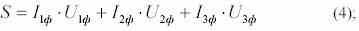- measure the number of revolutions of the disk of the meter of active electricity or the number of pulses of the light indicator (for electronic or microprocessor meters) N ​​for a time T (30-60 seconds is enough), then determine the active power by the formula: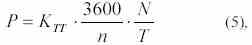where K TT is the transformation ratio of TT; n is the gear ratio of the counter. If there is a reactive energy meter, measure reactive power Q and calculate it using a formula similar to (5), and also determine the total power “by counters”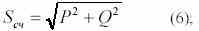- compare the values ​​of the total powers determined by formulas (4) and (6), which should coincide with the correct connection of the meter and serviceable elements of the measuring complex of electricity metering. In the absence of a reactive energy counter, the S and P values ​​are compared, which, if the meter is connected correctly, should not differ from each other by more than Cos ( f ) times. The smallest error introduced by the change in load is achieved with the "simultaneous" measurement of phase currents and the frequency of rotation of the disk of the meter. Using the proposed simple measurements and calculations, we can conclude that the meter is connected correctly and the metering complex for electricity metering is correct (current transformers, measuring circuits from CTs to meters, parallel meter windings, voltage circuits), as well as the amount of electricity that is not taken into account.